# Sag in a Transmission Line: What is it? (And How To Calculate it)

Contents

## What is Sag in a Transmission Line?

In a transmission line, sag is defined as the vertical difference in level between points of support (most commonly transmission towers) and the lowest point of the conductor. The calculation of sag and tension in a transmission line depends on the span of the overhead conductor.

Span having equal level supports (i.e. towers of the same height) is called level span. Conversely, when the span has unequal levels of support, this is known as unequal level span.

Consider a transmission line conductor AOB suspended freely between level supports A and B at the same level (equal span). The shape of the conductor is a parabola and the lowest point of the conductor is O.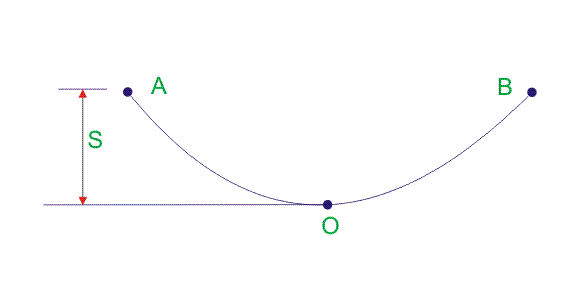In the above overhead conductor AOB, S is the sag when measured vertically.

## Why is Sag Mandatory in Transmission Line Conductors?

Sag is mandatory in transmission line conductor suspension. The conductors are attached between two supports with the perfect value of sag.

This is because it protects the conductor from excessive tension. In order to permit a safe level of tension in the conductor, conductors are not fully stretched; rather they are allowed to have sagged.

If the conductor is stretched fully during installation, wind exerts pressure on the conductor, hence the conductor gets a chance to be broken or detached from its end support. Thus sag is allowed to have during conductor suspension.

Some important points to note:

1. When the same leveled two supports hold the conductor, a bent shape arises in the conductor. Sag is very small with respect to the span of the conductor.
2. The Sag span curve is parabolic.
3. The tension in each point of the conductor acts always tangentially.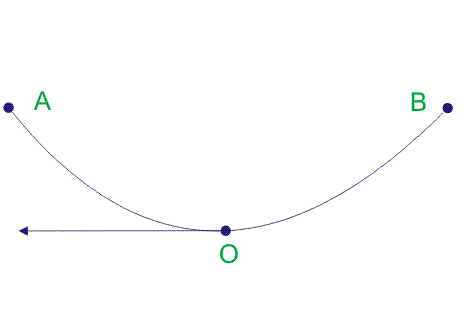1. Again the horizontal component of the tension of the conductor is constant throughout conductor length.
2. The tension at supports is nearly equal to the tension at any point in the conductor.

## How to Calculate Sag in a Transmission Line

When calculating sag in a transmission line, two different conditions need to be considered:

1. When supports are at equal levels
2. When supports are not at equal levels

The formula to calculate sag changes based on whether the support levels (i.e. the transmission towers holding up the overhead conductor) are at the same level.

Sag calculation for supports are at equal levels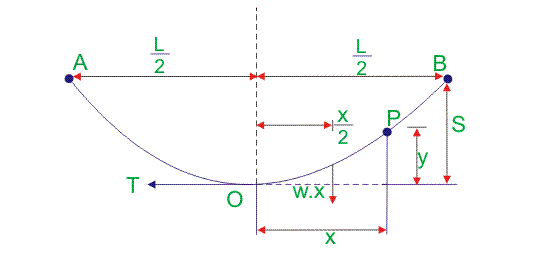Suppose, AOB is the conductor. A and B are points of supports. Point O is the lowest point and the midpoint.
Let, L = length of the span, i.e. AB
w is the weight per unit length of the conductor
T is the tension in the conductor.
We have chosen any point on the conductor, say point P.
The distance of point P from the Lowest point O is x.
y is the height from point O to point P.

Equating two moments of two forces about point O as per the figure above we get,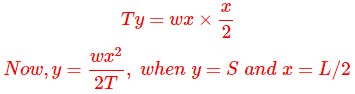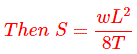Sag calculation for supports are at unequal levels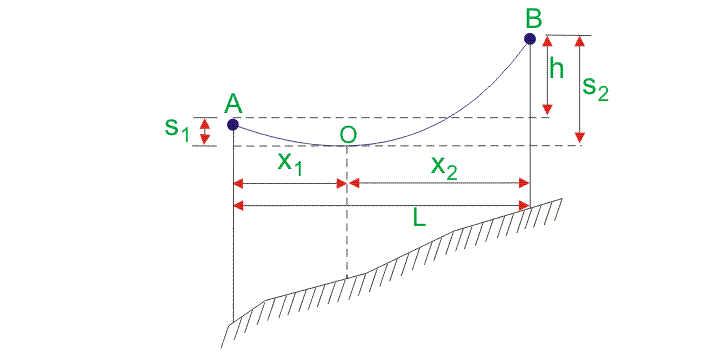Suppose AOB is the conductor that has point O as the lowest point.
L is the Span of the conductor.
h is the difference in height level between two supports.
x1 is the distance of support at the lower level point A from O.
x2 is the distance of support at the upper-level point B from O.
T is the tension of the conductor.
w is the weight per unit length of the conductor.
Now,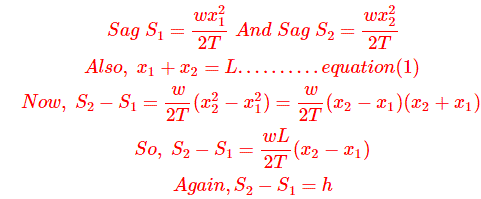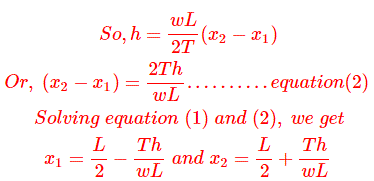So, having calculated the value of x1 and x2, we can easily find out the value of sag S1 and sag S2.

The above formula is used to calculate sag when the conductor is in still air and ambient temperature is normal. Hence the weight of the conductor is its own weight.

## What is the Effect of Ice and Wind on Sag?

Some of the effects of ice and wind on sag include:

• The weight per unit length of the conductor is changed when the wind blows at a certain force on the conductor and ice accumulate around the conductor.
• Wind force acts on the conductor to change the conductor self-weight per unit length horizontally in the direction of the airflow.
• Ice loading acts on the conductor to change the conductor self-weight per unit length vertically downward.
• Considering wind force and ice loading both at a time, the conductor will have a resultant weight per unit length.
• The resultant weight will create an angle with the ice loading down ward direction.

Let us assume, w is the weight of the conductor per unit length.
wi is the weight of ice per unit length
wi= density of ice × volume of ice per unit length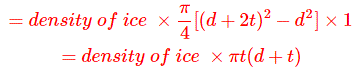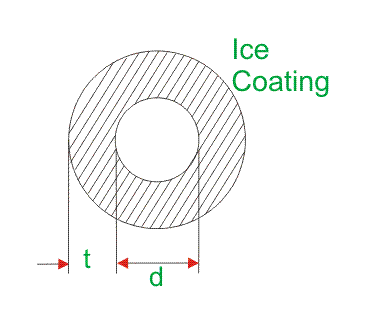ww is the force of wind per unit length
ww = wind pressure per unit area × projected area per unit length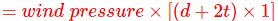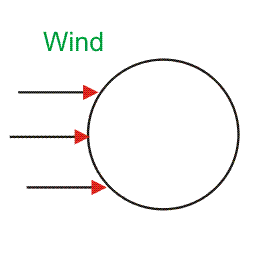So, the total weight of the conductor per unit length is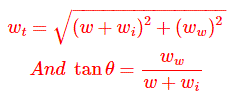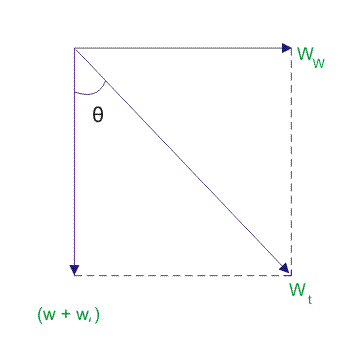The sag in the conductor is given by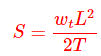So the vertical sag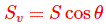Want To Learn Faster? 🎓
Get electrical articles delivered to your inbox every week.
No credit card required—it’s 100% free.